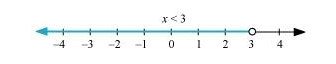# Solve the given inequality and show the graph of the solution on number line: 3x – 2 < 2x +1`
Question:

Solve the given inequality and show the graph of the solution on number line: $3 x-2<2 x+1$

Solution:

$3 x-2<2 x+1$

$\Rightarrow 3 x-2 x<1+2$

$\Rightarrow x<3$

The graphical representation of the solutions of the given inequality is as follows.